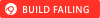## vsss-rs-std

Verifiable Secret Sharing Schemes for splitting, combining and verifying secret shares

### 5 releases

 3.0.0-pre4 Mar 20, 2023 Mar 14, 2023 Mar 13, 2023 Mar 10, 2023

#424 in Cryptography

Apache-2.0 OR MIT

93KB
2K SLoC

# Verifiable Secret Sharing SchemesThis crate provides various cryptography verifiable secret sharing schemes when the rust standard library is available.

This crate was separated from `vsss-rs` so both modes can be included in the same project.

• This implementation has not been reviewed or audited. Use at your own risk.
• This implementation targets Rust `1.51` or later.
• This implementation does not require the Rust standard library.
• All operations are constant time unless explicitly noted.

## NOTE if upgrading from Version 2

The standard mode has been split out into vsss-rs-std to enable both in the same project. In addition, the interfaces have been redesigned to be compatible with each other as well as serialization.

## Documentation

Verifiable Secret Sharing Schemes are using to split secrets into multiple shares and distribute them among different entities, with the ability to verify if the shares are correct and belong to a specific set. This crate includes Shamir's secret sharing scheme which does not support verification but is more of a building block for the other schemes.

This crate supports Feldman and Pedersen verifiable secret sharing schemes.

Feldman and Pedersen are similar in many ways. It's hard to describe when to use one over the other. Indeed both are used in distributed key generation.

Feldman reveals the public value of the verifier whereas Pedersen's hides it.

Feldman and Pedersen are different from Shamir when splitting the secret. Combining shares back into the original secret is identical across all methods and is available for each scheme for convenience.

This crate is no-std compliant and uses const generics to specify sizes.

This crate supports 255 as the maximum number of shares to be requested. Anything higher is pretty ridiculous but if such a use case exists please let me know.

Shares are represented as byte arrays. Shares can represent finite fields or groups depending on the use case. The first byte is reserved for the share identifier (x-coordinate) and everything else is the actual value of the share (y-coordinate).

When specifying share sizes, use the field size in bytes + 1 for the identifier.

### P-256

To split a p256 secret using Shamir

``````use vsss_rs::Shamir;
use ff::PrimeField;
use p256::{NonZeroScalar, Scalar, SecretKey};
use rand::rngs::OsRng;

fn main() {
let mut osrng = OsRng::default();
let sk = SecretKey::random(&mut osrng);
let nzs = sk.to_secret_scalar();
// 32 for field size, 1 for identifier = 33
let res = Shamir::<2, 3>::split_secret::<Scalar, OsRng, 33>(*nzs.as_ref(), &mut osrng);
assert!(res.is_ok());
let shares = res.unwrap();
let res = Shamir::<2, 3>::combine_shares::<Scalar, 33>(&shares);
assert!(res.is_ok());
let scalar = res.unwrap();
let nzs_dup =  NonZeroScalar::from_repr(scalar.to_repr()).unwrap();
let sk_dup = SecretKey::from(nzs_dup);
assert_eq!(sk_dup.to_bytes(), sk.to_bytes());
}
``````

### Secp256k1

To split a k256 secret using Shamir

``````use vsss_rs::Shamir;
use ff::PrimeField;
use k256::{NonZeroScalar, Scalar, SecretKey};
use rand::rngs::OsRng;

fn main() {
let mut osrng = OsRng::default();
let sk = SecretKey::random(&mut osrng);
let nzs = sk.to_secret_scalar();
let res = Shamir::<2, 3>::split_secret::<Scalar, OsRng, 33>(*nzs.as_ref(), &mut osrng);
assert!(res.is_ok());
let shares = res.unwrap();
let res = Shamir::<2, 3>::combine_shares::<Scalar, 33>(&shares);
assert!(res.is_ok());
let scalar = res.unwrap();
let nzs_dup = NonZeroScalar::from_repr(scalar.to_repr()).unwrap();
let sk_dup = SecretKey::from(nzs_dup);
assert_eq!(sk_dup.to_bytes(), sk.to_bytes());
}
``````

### BLS12-381

Feldman or Pedersen return extra information for verification using their respective verifiers

``````use vsss_rs::Feldman;
use bls12_381_plus::{Scalar, G1Projective};
use ff::Field;
use rand::rngs::OsRng;

fn main() {
let mut rng = OsRng::default();
let secret = Scalar::random(&mut rng);
let res = Feldman::<2, 3>::split_secret::<Scalar, G1Projective, OsRng, 33>(secret, None, &mut rng);
assert!(res.is_ok());
let (shares, verifier) = res.unwrap();
for s in &shares {
assert!(verifier.verify(s));
}
let res = Feldman::<2, 3>::combine_shares::<Scalar, 33>(&shares);
assert!(res.is_ok());
let secret_1 = res.unwrap();
assert_eq!(secret, secret_1);
}
``````

### Curve25519

Curve25519 is not a prime field but this crate does support it using `features=["curve25519"]` which is enabled by default. This feature wraps curve25519-dalek libraries so they can be used with Shamir, Feldman, and Pedersen.

Here's an example of using Ed25519 and x25519

``````use curve25519_dalek::scalar::Scalar;
use ed25519_dalek::SecretKey;
use vsss_rs::{Shamir, WrappedScalar};
use rand::rngs::OsRng;
use x25519_dalek::StaticSecret;

fn main() {
let mut osrng = rand::rngs::OsRng::default();
let sc = Scalar::random(&mut osrng);
let sk1 = StaticSecret::from(sc.to_bytes());
let ske1 = SecretKey::from_bytes(&sc.to_bytes()).unwrap();
let res = Shamir::<2, 3>::split_secret::<WrappedScalar, OsRng, 33>(sc.into(), &mut osrng);
assert!(res.is_ok());
let shares = res.unwrap();
let res = Shamir::<2, 3>::combine_shares::<WrappedScalar, 33>(&shares);
assert!(res.is_ok());
let scalar = res.unwrap();
assert_eq!(scalar.0, sc);
let sk2 = StaticSecret::from(scalar.0.to_bytes());
let ske2 = SecretKey::from_bytes(&scalar.0.to_bytes()).unwrap();
assert_eq!(sk2.to_bytes(), sk1.to_bytes());
assert_eq!(ske1.to_bytes(), ske2.to_bytes());
}
``````

Either `RistrettoPoint` or `EdwardsPoint` may be used when using Feldman and Pedersen VSSS.